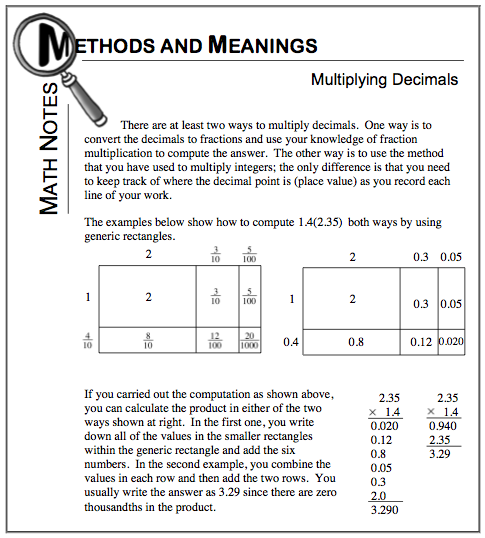### Home > CC1 > Chapter Ch6 > Lesson 6.2.4 > Problem6-110

6-110.

Evaluate the expressions below for the given values of the variables. Homework Help ✎

For the expression below, you can substitute the values given for each variable and then simplify the expression.
It is important to remember that 2p really means two p's or (2) x (p).
2p + 8 for p = 4
2(4) + 8 = 8 + 8 = 16
And, remember to follow the Order of Operations!

1. $6j−3\ \text{for}\ j=4$

Be sure to show all of your work!
This way you will be less likely to make mistakes.

21

2. $b(b)+5 \text{ for } b=3$

If the variable is substituted here, the expression becomes 3(3) + 5.
Can you simplify it now?

14

3. $8+4k \text{ for } k=3.5$

• Remember to follow the rules of multiplication. Refer to the Math Box below for help with multiplying decimals.